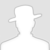FreeBuf.COM网络安全行业门户，每日发布专业的安全资讯、技术剖析。FreeBuf+小程序

Dance In Heap（二）：一些堆利用的方法（上）2017-10-27 09:00:45

*本文原创作者：hellowuzekai，属于FreeBuf.COM，禁止转载。

## 相关链接：

Dance In Heap（四）：一些堆利用的方法（下）

## 0x00 前面的话

``````0x01 Use After Free
0x02 Unlink
0x03 unsortedbin attack
0x04 小结
``````

## 0x01 Use After Free

``````#include<stdio.h>
#include<stdlib.h>
struct shell {
void (*getshell)();
};
struct data {
int data;
};
void test_getshell(){
printf("I get the shell\n");
}

int main () {
struct shell *p;
p = (struct shell*)malloc(sizeof(struct shell));

p->getshell = test_getshell;
free(p);
struct data *q;
q = (struct data*)malloc(sizeof(struct data));
q->data = 1234;
p->getshell();
return 0;
}
``````

``````Legend: code, data, rodata, value
Stopped reason: SIGSEGV
0x00000000000004d2 in ?? ()
``````

## 0x02 unlink

unlink漏洞想必大家都不陌生，在前面我们提到过，系统通过 unlink 宏将 free chunk 从链表中取出，但是我在这里强调一下，并非所有从链表中取出 chunk 的操作都利用到了 unlink 宏，要知道，我们在 malloc 时，也多次将 chunk 从 bin 中取出，我想结合部分源码（只截取了取出部分的代码）来强调一下 unlink 的使用状况。

1. 从 fastbin 中取出 chunk

``````mfastbinptr* fb = &fastbin (av, idx);
victim = *fb;
*fb = victim->fd;
``````
2. 从 unsortedbin 中取出 chunk

``````victim = unsorted_chunks(av)->bk
bck = victim->bk;
unsorted_chunks(av)->bk = bck;
bck->fd = unsorted_chunks(av);
``````
3. 从 unsortedbin 向 smallbin 转移 chunk

``````if (in_smallbin_range(size)) {
victim_index = smallbin_index(size);
bck = bin_at(av, victim_index);
fwd = bck->fd;
``````
4. 从 unsortedbin 向 largebin 转移 chunk

``````mark_bin(av, victim_index);
victim->bk = bck;
victim->fd = fwd;
fwd->bk = victim;
bck->fd = victim;
``````
5. 从 smallbin 中取出 chunk

``````idx = smallbin_index(nb);
bin = bin_at(av,idx);
victim = last(bin);
bck = victim->bk;
bin->bk = bck;
bck->fd = bin;
``````
6. 从 largebin 中取出 chunk

``````unlink(victim, bck, fwd);
``````
7. 合并 fastbin 中 chunk 并加入到 unsortedbin 中(单向链表，bk指针需要获取)

``````prevsize = p->prev_size;
size += prevsize;
p = chunk_at_offset(p, -((long) prevsize));115
unlink(p, bck, fwd);
......
size += nextsize;
unlink(nextchunk, bck, fwd);
``````

我们发现不仅仅在 free 时进行向前向后合并时使用 unlink 宏，在 malloc 时也会有零星的 unlink 使用，而且一定要注意，上面的除了6、7外，在进行取出 chunk 操作时，并没有进行 unlink，所以在对这一部分进行漏洞利用时，不需要考虑 unlink 的检查。

``````#define unlink(P, BK, FD) {
FD = P->fd;
BK = P->bk;
if (__builtin_expect (FD->bk != P || BK->fd != P, 0))
malloc_printerr (check_action, "corrupted double-linked list", P);
else {
FD->bk = BK;
BK->fd = FD;
``````

``````a = malloc(0x20)
b = malloc(0x20) // b.size = 0x20 + chunk_header | inuse(0x01) == 0x31
a[0x20+4] = 0x30 // 覆盖 inuse 位
free(b) // 检查inuse位，发现 a 为 free，执行 unlink，合并两个 chunk
``````

``````    P->bk->fd == P // [P+0x18]+0x10 == P [P+0x18] == P-0x10
P->fd->bk == P // [P+0x10]+0x18 == P [P+0x10] == P-0x18
``````

``````    FD->bk = BK   *P = P - 0x10(0x8)
BK->fd = FD   *P = P - 0x18(0xc) // 这一步覆盖上一步
``````

``````--------|-------|
b   |       |----
--------|-------|    |
····· |       |    |
--------|-------|    |
b-0x18 |       |<---
--------|-------|
``````

``````--------|-------|      |-------|
b   |address|----> |  写入 |
--------|-------|      |-------|
····· |       |
--------|-------|
b-0x18 | AAAA  |
--------|-------|
``````

## 0x03 unsortedbin attack

``````p = malloc(0x400)
malloc(0x200)
``````

``````free(p)
p = 0xdeadbeef-0x10 // 任意地址 - 0x10
malloc(0x400)
``````

``````victim = unsorted_chunks(av)->bk // victim为free掉的p
bck = victim->bk;  // bck 为 任意地址 -0x10
unsorted_chunks(av)->bk = bck; // 调整链表
bck->fd = unsorted_chunks(av); //  任意地址 -0x10 + 0x10 = unsortedbin
``````

## 0x04 小结

*本文原创作者：hellowuzekai，属于FreeBuf.COM，禁止转载。

# 内存保护 # 堆利用

+ 收入我的专辑• 0 文章数
• 0 评论数
• 0 关注者